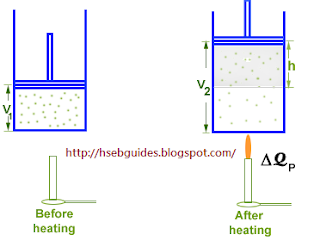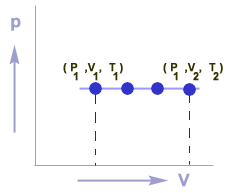## TyroCity

Physics XI Notes for Physics Notes

Posted on • Updated on

# First Law Of Thermodynamics

Thermodynamics
Thermodynamics is the branch of science which deals with the interconversion of heat energy and mechanical energy. All those problems that are related to the interconversion of heat energy and work done are studied in thermodynamics. In thermodynamics we discuss different cycles such as Carnot cycle, Rankine cycle, Otto cycle, diesel cycle, refrigerator, IC engines, EC engines, Compressors, turbines and air conditioners.

First law of thermodynamics
Statement:

“During any process total energy of a system and its surroundings is constant.”
Or
“It is impossible to construct a machine which performs work
continuously with taking energy from an external source.”
Or
“Energy can neither be created nor destroyed but it can be
converted from one form of energy to another form of energy.”

Mathematical representation
Let a system absorbs DQ amount of heat energy. Addition of heat energy increases the internal energy of system from U1 to U2 and some useful work is also performed by the system.
Increase in internal energy is given by:
DU = U1 – U2
and
work done is DW
According to the first law of thermodynamics:
DQ = DU+ DW

Sign convention:
DQ = positive if heat is added to a system
DQ = negative if heat is released from a system
DW = positive if work is done by the system
DW = negative if work is done on the system
APPLICATIONS OF THE FIRST LAW OF THERMODYNAMICS
Heat can be supplied to a thermodynamic system under the following conditions:

• Isobaric process
• Isochoric process
• Isothermal process

Isobaric process
A thermodynamic process in which pressure of the system remains constant during the supply of heat is called an isobaric process.

Explanation:Consider a cylinder fitted with a frictionless piston. The piston is free to move in the cylinder. An ideal gas is enclosed in the cylinder.

Let the initial volume of the system is V1 and initial internal energy is U1. Let DQP the gas is heated from

T1 K to T2 K. Addition of heat causes the following changes in the system:
Internal energy increases from U1 to U2.
Volume of the system increases from V1 to V2.
Temperature increases from T1 K to T2 K.
Work (DW) is done by the gas on the piston.
According to the first law of
thermodynamics:
DQ = DU+ DW
But DW = PDV
Thus,
DQP = DU+ PDV
As
DV = (V2 – V1)
DQP = DU+ P (V2 – V1)

Graphical representation
Graph between P & V for an isobaric process is a straight line which is parallel to V-axis.Advantages of first law of thermodynamics:

1. Total energy of the universe remains constant.
2. Different forms of energy are inter-convertible.
3. When one form of energy disappears, an equivalent amount of energy in another form appears.

Disadvantages of first law of thermodynamics:

1. It doesn’t tell the extent and direction of the convertibility of one form of energy to another.
2. It doesn’t tell why chemical reactions do not proceed to completion.
3. It doesn’t tell why natural processes are unidirectional.
4. It doesn’t explain the feasibility and spontaneity of a process.
5. It says the equivalency of work and heat. But it has been observed that the work can be completely transformed into heat but heat cannot be transformed into work without permanent change in the system or surrounding which is not explained by the law.